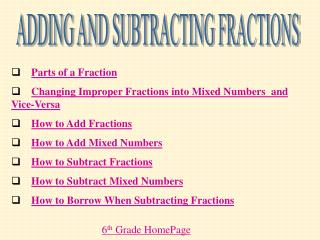DownloadDownload PresentationDownload Presentation- - - - - - - - - - - - - - - - - - - - - - - - - - - E N D - - - - - - - - - - - - - - - - - - - - - - - - - - -
##### Presentation Transcript

1. ADDING AND SUBTRACTING FRACTIONS • Parts of a Fraction • Changing Improper Fractions into Mixed Numbers and Vice-Versa • How to Add Fractions • How to Add Mixed Numbers • How to Subtract Fractions • How to Subtract Mixed Numbers • How to Borrow When Subtracting Fractions 6th Grade HomePage

2. PARTS OF A FRACTION The top number of a fraction is the numerator. The bottom number of a fraction is the denominator. 3 4 6th Grade HomePage

3. QUIZ MIXED NUMBERS AND IMPROPER FRACTIONS 6th Grade HomePage To change a mixed number into an improper fraction get MAD…Multiply – Add – Put over Denominator 4 ½ 4 x 2 = 8 8 + 1 = 9 9 2 To change an improper fraction into a mixed number divide the numerator by the denominator; whatever is left over is the new numerator…keep the same denominator. For Example: 11 4 11 divided 4 equals 2; there is 3 left over. 34 2

4. Quiz: Changing Mixed Numbers and Improper Fractions • Change the following into Improper Fractions.REVIEW • 3 ½ • 5 5/8 • 12 1/3 • 15 ¾ • Change the following into Mixed Numbers.REVIEW • 40/13 • 100/9 • 4/3 • 4. 9/2 CHECK 6th Grade HomePage

5. ANSWERS: Changing Mixed Numbers and Improper Fractions • Change the following into Improper Fractions. • 1. 3 ½ = 7/2 • 5 5/8 = 45/8 • 12 1/3 = 37/3 • 15 ¾ = 63/4 • Change the following into Mixed Numbers. • 40/13 = 3 1/13 • 100/9 = 11 1/9 • 4/3 = 1 1/3 • 4. 9/2 = 4 ½ 6th Grade HomePage

6. 15 20 3 4 15_ 5 = 5 = x + 4 = 4 = 4 20 x ADDING FRACTIONS When adding fractions you MUST have a common denominator! Find the smallest number that both 4and 5 will go into. This number is 20. Then multiply both the numerator and denominator to get to 20. 19 20 Once you have a common denominator, then add! 6th Grade HomePage

7. QUIZ ADDING MIXED NUMBERS 3 3 • ½ • 5 1/3 X X 6 5 3/6 2/6 2 2 + 11 5/6 You MUST have a common denominator to add fractions! Find the least common multiple of 3 and 2. This number is 6. Once you have the common denominator, add the fractions, then add the whole numbers! 6th Grade HomePage

8. X X X X 1 1 2 2 QUIZ SUBTRACTING FRACTIONS 5 6 2 3 5 6 4 6 Find the least common multiple for 3 and 6. (In this case, 6) Then multiply! 1 6 This is your answer! To subtract fractions you must have a common denominator! 6th Grade HomePage

9. Answers: Adding Fractions • 3 1/5 2. 2 ½ • + 4 ¼ + 1 ¾ • 5 1/6 4. 1/3 • + 2 2/3 1/4 • + 1/2 CHECK Review 6th Grade HomePage

10. QUIZ: Adding Fractions • 3 1/5 2. 2 ½ • + 4 ¼ + 1 ¾ • 7 9/20 3 5/4 = 4 1/4 • 5 1/6 4. 1/3 • + 2 2/3 1/4 • 7 5/6 + 1/2 • 13/12 = 1 1/12 Review 6th Grade HomePage

11. QUIZ SUBTRACTING MIXED NUMBERS 2 2 1 1 • 2/4 • ¼ • ½ • ¼ X X • ¼ To subtract fractions you must have a common denominator! Find the least common multiple of 4 and 2. This number is 4. ANSWER! 6th Grade HomePage

12. 2/4 • 2 3/4 SUBTRACTING FRACTIONS WHILE BORROWING + 4/4 2 2 1 1 4 2/4 2 3/4 • 6/4 • 2 3/4 • ½ • ¾ X X First…Find a common denominator. 2 3/4 You cannot subtract 3 from 2, so you MUST borrow! ANSWER! Find the least common multiple of 4 and 2. This number is 4. 6th Grade HomePage

13. Quiz: SUBTRACTING FRACTIONS 6th Grade HomePage • ½ 2. 3 ¾ • - ¼ - 1 ½ • 4 1/5 4. 5 • - 1 1/2 - 3 ¾ CHECK Review Subtracting Review Subtracting While Borrowing

14. ANSWERS: SUBTRACTING FRACTIONS 6th Grade HomePage • ½ 2. 3 ¾ • - ¼ - 1 ½ • ¼ 2 ¼ • 4 1/5 4. 5 • - 1 1/2 - 3 ¾ • 2 7/10 1 ¼ Review Subtracting Review Subtracting While Borrowing